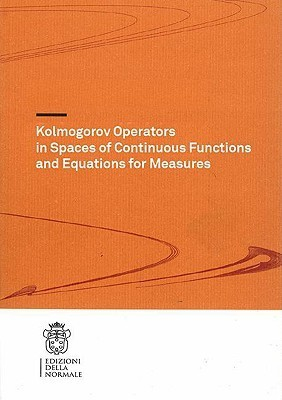Home » Kolmogorov Operators in Spaces of Continuous Functions and Equations for Measures by Luigi Manca# Kolmogorov Operators in Spaces of Continuous Functions and Equations for Measures

## Luigi Manca

Published February 1st 2009
ISBN : 9788876423369
Paperback
127 pages
Book Rating:Enter the sum

 About the Book The book is devoted to study the relationships between Stochastic Partial Differential Equations and the associated Kolmogorov operator in spaces of continuous functions.In the first part, the theory of a weak convergence of functions is developed inMoreThe book is devoted to study the relationships between Stochastic Partial Differential Equations and the associated Kolmogorov operator in spaces of continuous functions.In the first part, the theory of a weak convergence of functions is developed in order to give general results about Markov semigroups and their generator.In the second part, concrete models of Markov semigroups deriving from Stochastic PDEs are studied. In particular, Ornstein-Uhlenbeck, reaction-diffusion and Burgers equations have been considered. For each case the transition semigroup and its infinitesimal generator have been investigated in a suitable space of continuous functions.The main results show that the set of exponential functions provides a core for the Kolmogorov operator. As a consequence, the uniqueness of the Kolmogorov equation for measures has been proved.